Ex 10.4

Chapter 10 Class 11 Conic Sections
Serial order wise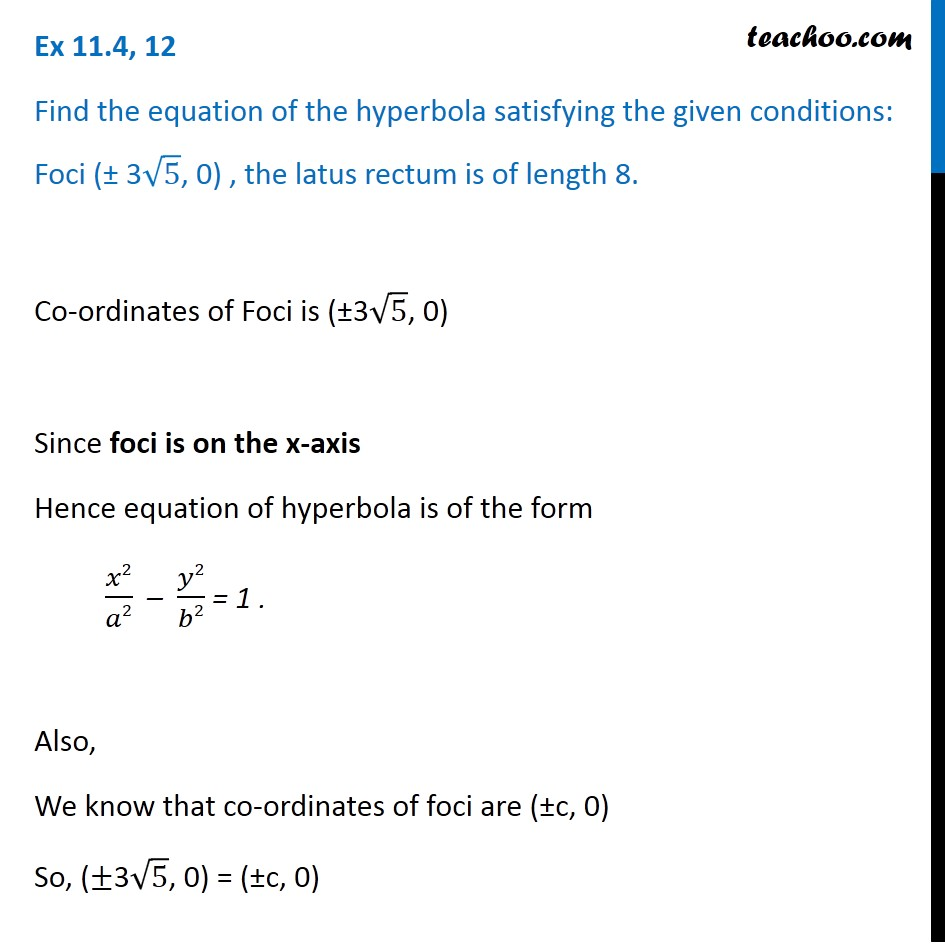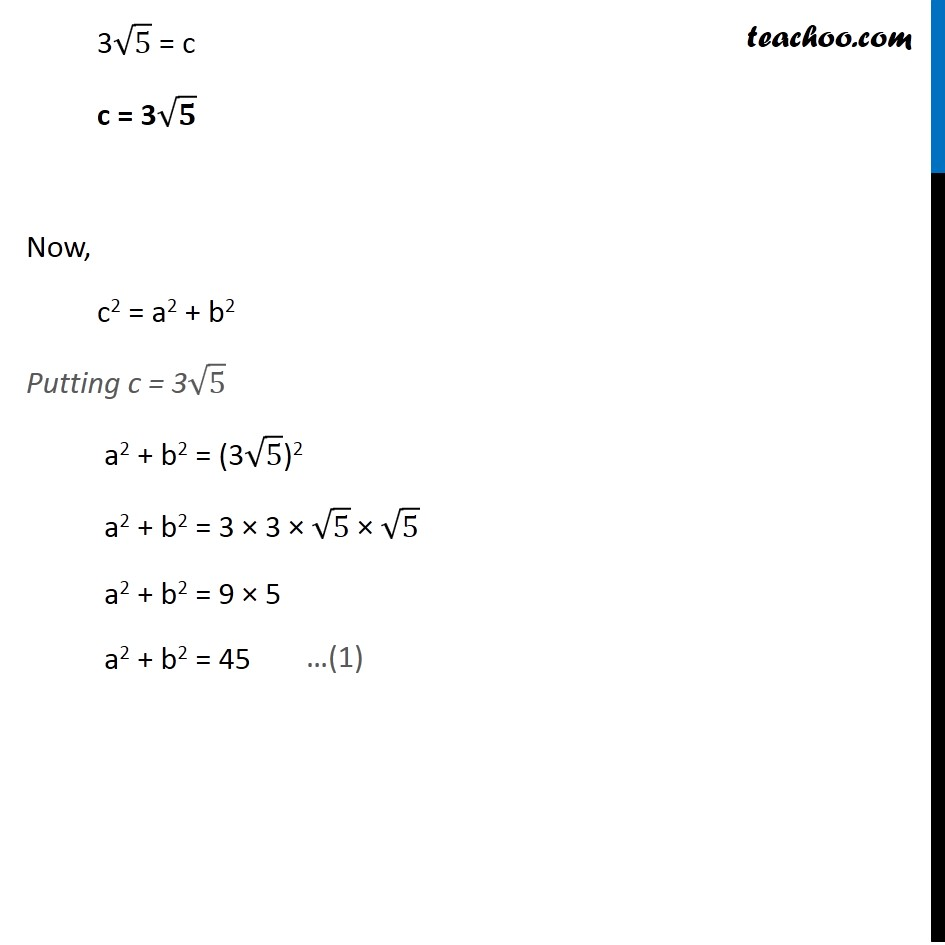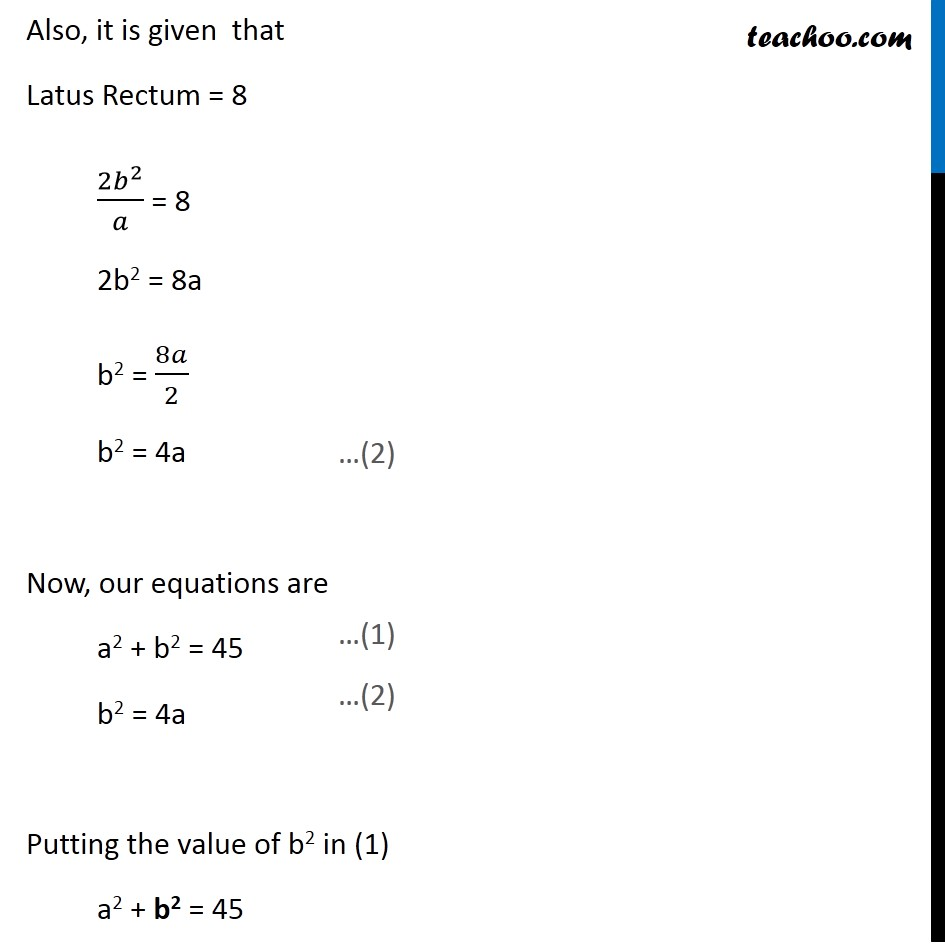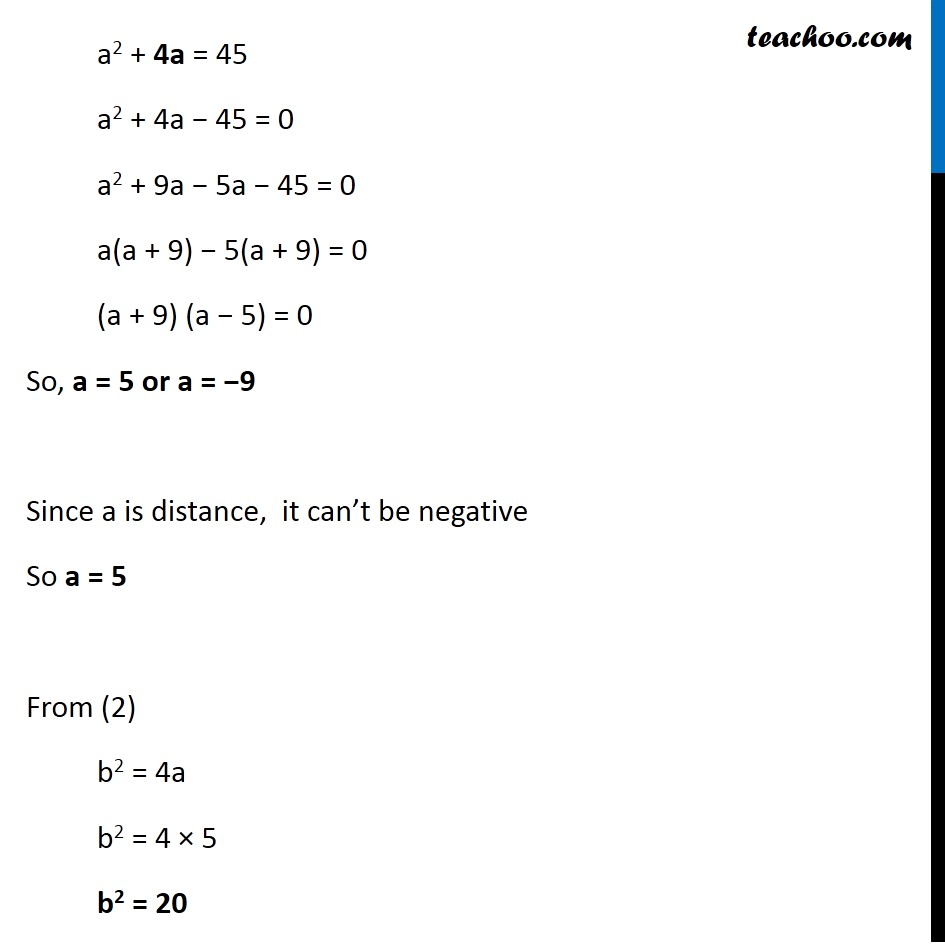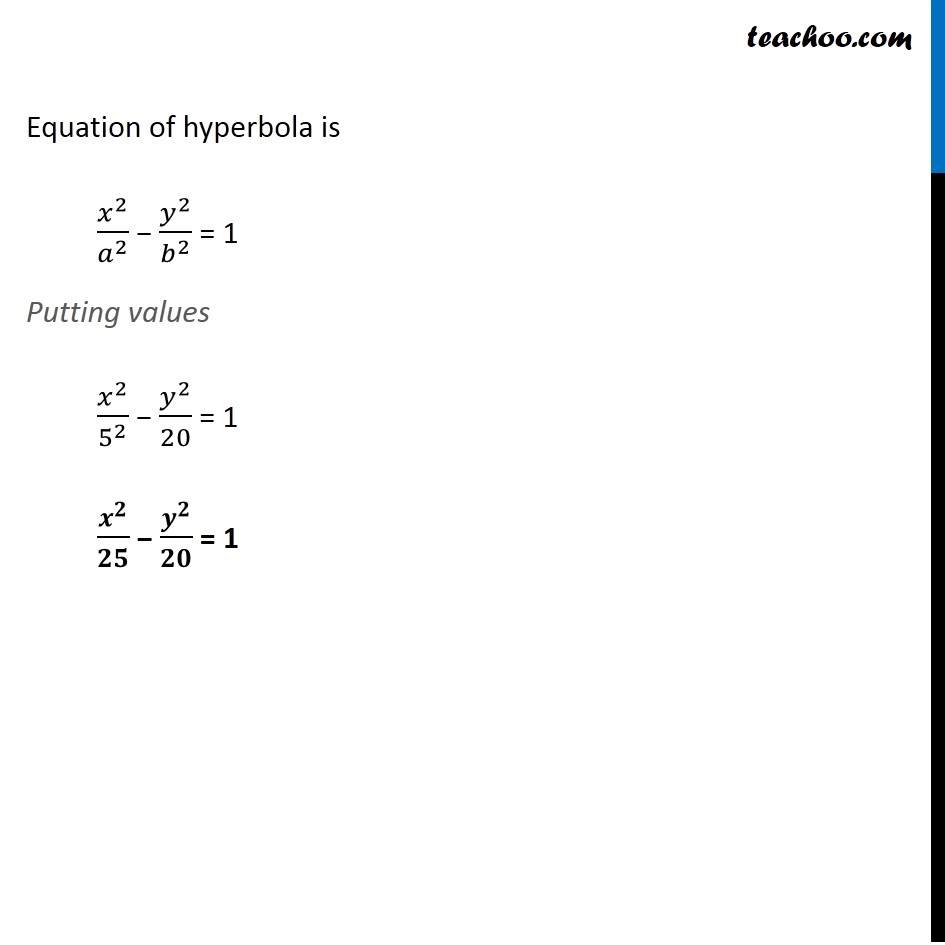Learn in your speed, with individual attention - Teachoo Maths 1-on-1 Class

### Transcript

Ex 10.4, 12 Find the equation of the hyperbola satisfying the given conditions: Foci (± 3√5, 0) , the latus rectum is of length 8. Co-ordinates of Foci is (±3√5, 0) Since foci is on the x-axis Hence equation of hyperbola is of the form 𝑥2/𝑎2 – 𝑦2/𝑏2 = 1 . Also, We know that co-ordinates of foci are (±c, 0) So, (±3√5, 0) = (±c, 0) 3√5 = c c = 3√𝟓 Now, c2 = a2 + b2 Putting c = 3√5 a2 + b2 = (3√5)2 a2 + b2 = 3 × 3 × √5 × √5 a2 + b2 = 9 × 5 a2 + b2 = 45 Also, it is given that Latus Rectum = 8 (2𝑏^2)/𝑎 = 8 2b2 = 8a b2 = 8𝑎/2 b2 = 4a Now, our equations are a2 + b2 = 45 b2 = 4a Putting the value of b2 in (1) a2 + b2 = 45 a2 + 4a = 45 a2 + 4a − 45 = 0 a2 + 9a − 5a − 45 = 0 a(a + 9) − 5(a + 9) = 0 (a + 9) (a − 5) = 0 So, a = 5 or a = −9 Since a is distance, it can’t be negative So a = 5 From (2) b2 = 4a b2 = 4 × 5 b2 = 20 Equation of hyperbola is 𝑥^2/𝑎^2 − 𝑦^2/𝑏^2 = 1 Putting values 𝑥^2/5^2 − 𝑦^2/20 = 1 𝒙^𝟐/𝟐𝟓 − 𝒚^𝟐/𝟐𝟎 = 1